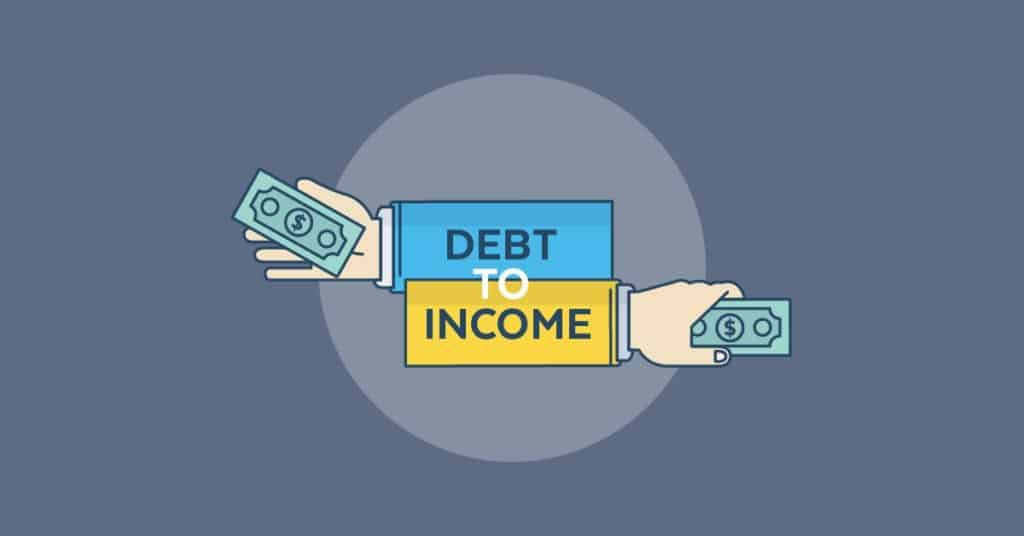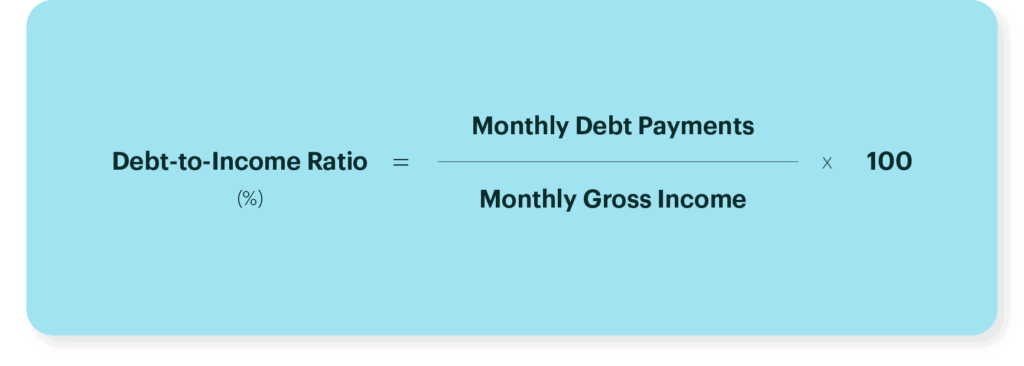# What Is Debt To Income Ratio? A Complete Guide## Debt To Income Ratio Meaning?

A Debt-to-income ratio is a ratio that the banks calculate and take into account to examine your loan eligibility via your gross monthly income. Here, the higher the DTI ratio, the lower the chances of you getting approved for a fresh loan

In simple words, prior to the bank approving your loan application, they would examine your repayment capacity via calculating the debt-to-income (DTI) ratio. Mostly calculated in percentage, the DTI ratio is obtained simply from your net monthly debt payments (such as credit card bills, education loans, auto loans, personal loans, etc), by your gross monthly income.

## Formula To Calculate Your Debt To Income - How To Calculate Debt Ratio To Income?The formula to calculate the debt to income ratio is:

DTI = Net debt payments / Net income

Note – As the result is viewed in a decimal number, one would need to multiply the result by 100 in order to get the DTI in percentage terms.

 Debt-to-income DTI Ratio Calculation DTI = (Net debt payments/Net income) x 100 DTI ratio in India Maximum of 40% How to improve DTI Increase in the salary amount Decrease in the total monthly EMI

For instance – Suppose Tom earns a monthly income of about Rs 80,000. From the total, Rs 25,000 is spent on the EMI for his car loan and Rs 15,000 for his educational loan. This means, he spends about half of his income, that is, Rs 40,000 each month in simply repaying the debts as mentioned above. In this example, if you are to calculate his DTI ratio, you will need to divide the monthly income (Rs 80,000) with his monthly debt payments (Rs 40,000) and multiply the results by 100, then the DTI ratio would be 50%.

## What Is The Acceptable Debt to Income Ratio Cap For Indian Banks?

In India, the debt to income ratio of 40% is considered to be the highest for a borrower to get a new loan. A lender would always prefer a loan applicant with less DTI ratio.

## What Is The Ideal Debt To Income Ratio For Home Loan?

As soon as an applicant applies for a home loan, the lender would consider the finances, such as the credit history, gross monthly income, and the down payments (if any). The DTI is the ratio that the lender looks into to know how much you can afford for the house in question.

Generally, the lenders prefer a DTI ratio that is lower than 36% with a maximum of 28% debt going towards servicing your mortgage. For instance, if Sam has a gross income is Rs 4,000 per month, then the maximum amount for monthly mortgage-related payments at 28% would be Rs 1,120, that is Rs 4,000 x 0.28 = Rs 1,120.

Besides, the lender would also look at the total debts that must not exceed 36%. However, in most cases, 43% is considered to be the highest ratio that a borrower can have to qualify for a home loan.

Note – In case the lender denies the home loan application, it would be due to your monthly expenses towards housing being too high as compared to your income.

## How Can An Individual Improve A High DTI Ratio?

You can easily improve your DTI ratio in two simple ways:

1. If there is an increase in your salary
2. If there is a decrease in the existing monthly payments

Where, (1) can be obtained if you choose to switch jobs or receive a hike in your salary, and (2) If you are able to get your existing loans refinanced, effectively lowering the monthly EMI payment while increasing the loan tenure.

For instance, if Tom lowers his education loan EMI up to Rs 10,000 and the car loan EMI up to 20,000, then in that case his DTI ratio would be 37.5%. The lender in this case would be willing to approve your request for debt.

## Key Facts About The DTI ratio?

The higher the DTI ratio the lower the chances of getting the loan – As already mentioned in the article, if the bank reviews a large part of the monthly income being spent on repaying the previous debt, the request for a loan might not get accepted/entertained.

Try and keep the DTI ratio low – In order to increase the chances of getting the loan amount accepted, a borrower must try to limit their DTI ratio. The lower the DTI ratio, the higher the chances of getting a fresh loan.

Maintain a good credit score – A credit score is another way to determine the worthiness of a borrow of repaying fresh loans. Therefore, there is a possibility to have a good credit score despite the high DTI ratio, as it is generated via timely payment of the debts. Haven said that a high DTI ratio and a good credit score don’t guarantee to get a new loan.

## In Conclusion: Here Is A Quick Recap About The DTI Ratio

1. The debt to income ratio is expressed in percentage terms
2. Debt to income is calculated on a monthly basis
3. The banks cap the DTI limit to about 40%

### 1. What is the upper DTI ratio limit for Indian banks?

Banks in India do not approve a loan request for a fresh loan if they notice an individual using more than 40% of their net income to repay existing debts.

### 2. Can I get a home loan if 50% of my income is used in repaying other loans?

If 50% of the income is used in repaying other loans, then the banks might not approve the loan request.

### 3. What bills are included in the debt to income ratio?

A lender only looks into the monthly debt payments in order to determine if the borrower is capable of repaying the loan by determining the debt-to-income ratio.

### 4. What is the maximum monthly debt to income DTI ratio to qualify for a standard qualified mortgage?

Generally, the highest DTI ratio for a borrower to qualify for a mortgage is 43%. However, ideally, most lenders prefer a DTI lower than 36%, with no more than 28% of that debt going towards servicing a mortgage or rent payment.#### Property Geek

Share now!Here's more to look into!ENQUIRY